# Financial Ratios Practice Calculations

Now that you have learned about 15 of the more common financial ratios, we want you to experience calculating them by using the amounts in a corporation's financial statements. This will deepen your understanding and will help your retention for future use.

The financial ratios to be calculated are arranged in the same order as they were discussed above:

1. Financial ratios using amounts from the balance sheet
2. Financial ratios using amounts from the income statement
3. Financial ratios using amounts from the balance sheet and income statement
4. Financial ratio using amounts from the statement of cash flows

### Calculating the Ratios Using Amounts from the Balance Sheet

You will be using the following balance sheet to calculate the first group of financial ratios: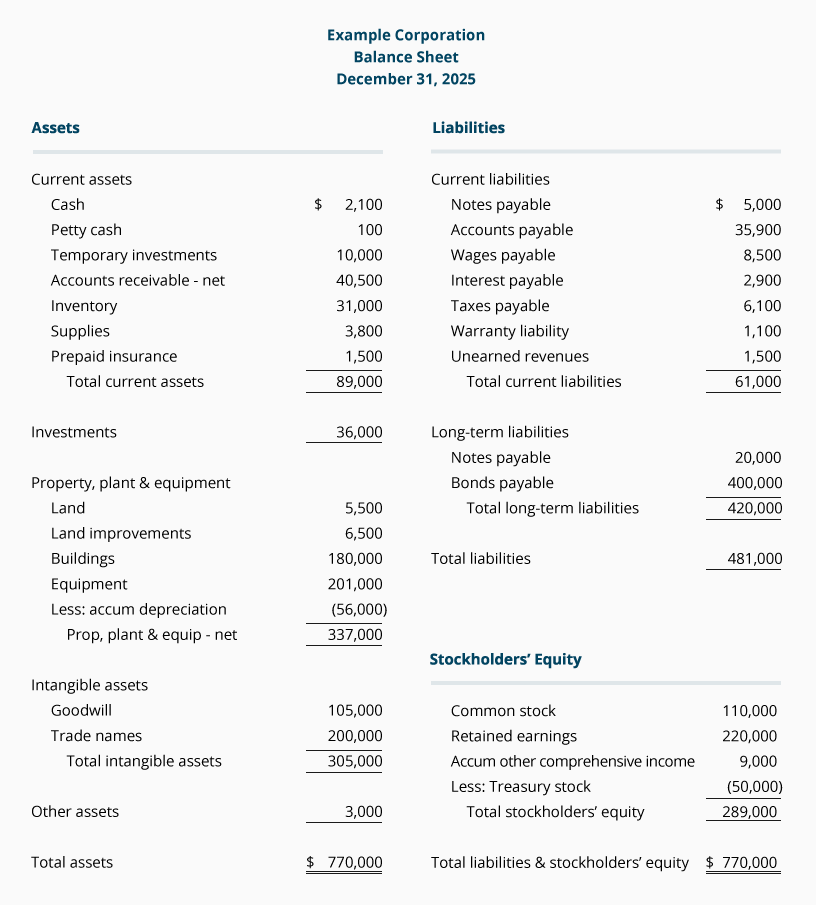Calculations of Working Capital, Current Ratio, and Quick Ratio
Use the amounts in Example Corporation's balance sheet (above) to calculate the following financial ratios:

• Working capital as of December 31, 2022: \$____________

• Current ratio as of December 31, 2022: ______: 1

• Quick ratio as of December 31, 2022: ______: 1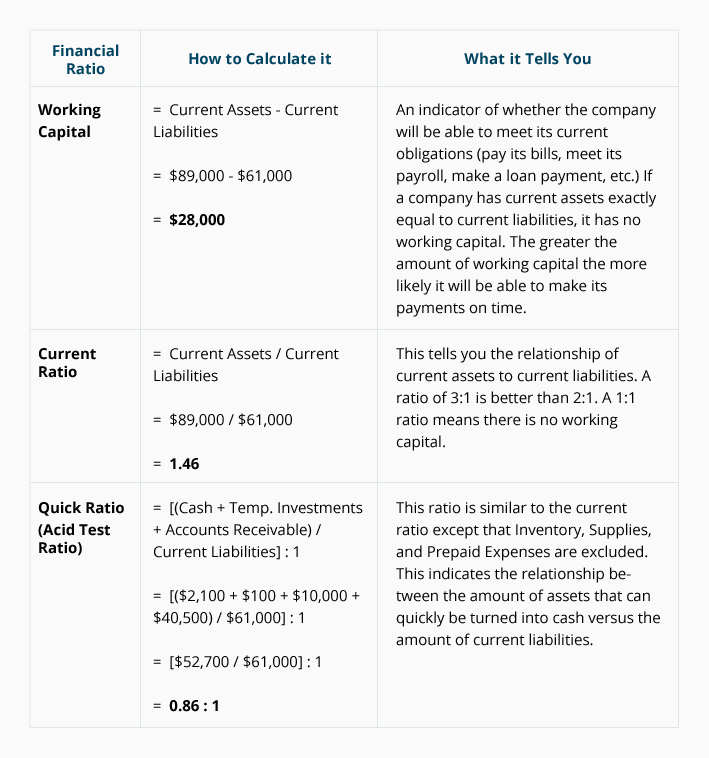Calculations of Debt to Equity and Debt to Total Assets Ratios
Continue using the amounts in Example Corporation's balance sheet to compute these two financial ratios:

• Debt to equity ratio as of December 31, 2022: __________

• Debt to total assets ratio as of December 31, 2022: _________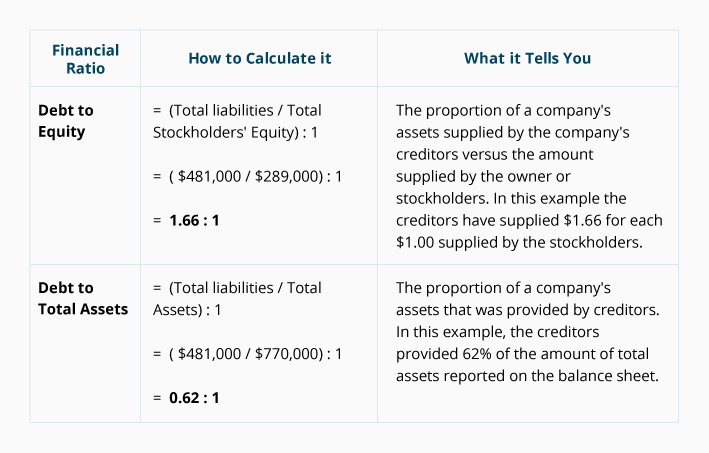### Calculating the Ratios Using Amounts from the Income Statement

The following income statement for Example Corporation should be used to calculate the four financial ratios which appear beneath it: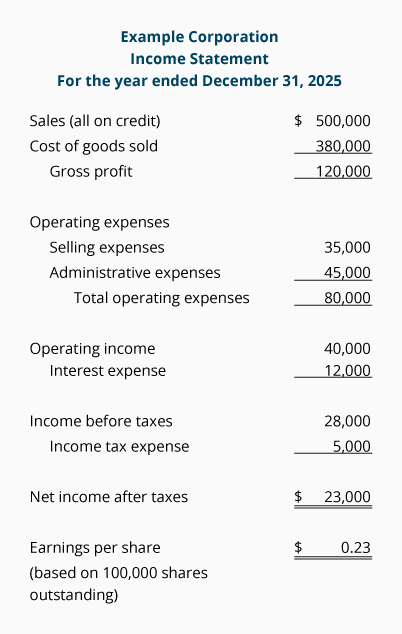Calculations of Gross Margin, Profit Margin, Earnings Per Share, and Times Interest Earned
Use the amounts in Example Corporation's income statement (above) to compute these financial ratios:

• Gross margin for the year ended December 31, 2022: __________

• Profit margin for the year ended December 31, 2022: __________

• Earnings per share for the year ended December 31, 2022: ________

• Times interest earned for the year ended December 31, 2022: ________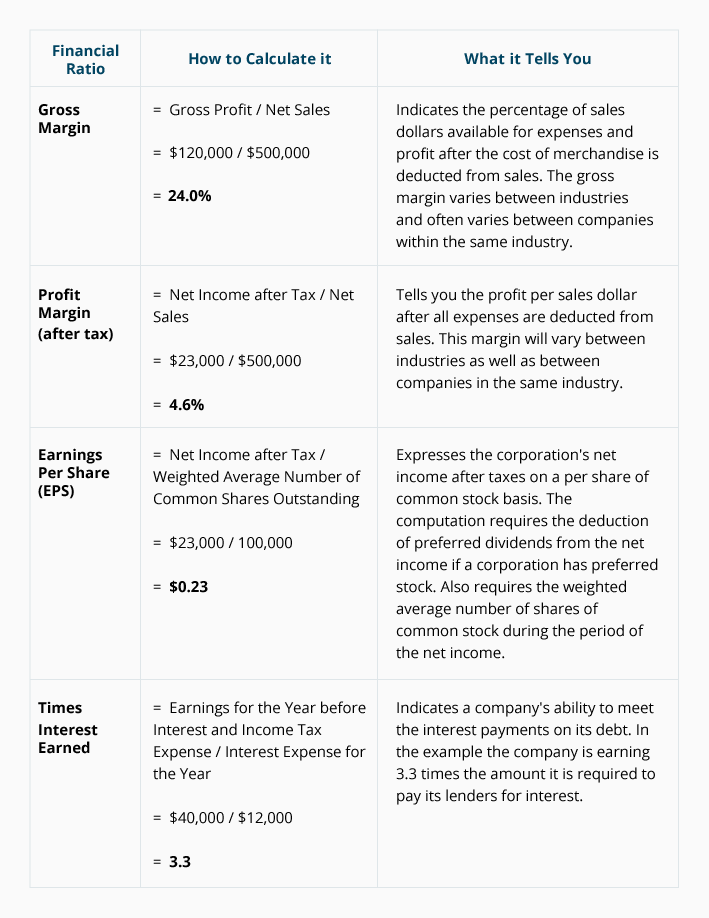### Calculating the Ratios Using an Amount from the Balance Sheet and the Income Statement

Financial ratios such as the "turnover" ratios and the "return on" ratios will need 1) an amount from the annual income statement, and 2) an average balance sheet amount.

An average balance sheet amount is needed since the balance sheet reports the amount for only the final moment of the accounting year. For the required calculations that follow, we indicate the average balance sheet amount.

Calculations of the Ratios: Receivables Turnover, Day's Sales in Receivables, Inventory Turnover, Days' Sales in Inventory, Return on Stockholders' Equity
Calculate the following ratios using Example Corporation's income statement and our calculated average balance sheet amounts* which are included in the following questions:

• Receivables turnover ratio for the year 2022 assuming the average* accounts receivable balance during the year was computed to be \$42,000: __________

• Days' sales in receivables (average collection period) for the year 2022: __________

• Inventory turnover ratio for the year 2022 assuming that the average* inventory balance during the year was computed to be \$30,000: _________

• Days' sales in inventory (days to sell) for the year 2022: _________

• Return on stockholders' equity for the year 2022 assuming that the average* stockholders' equity balance during the year was computed to be \$278,000: _________

*We are providing the average balance sheet amounts based on the corporation's internal records for all days in 2022. Using only the balance sheet amount from the final moment of 2022 (or the average of the final moment of 2021 and 2022) may not be typical of the balance sheet amount for all 365 days in the year.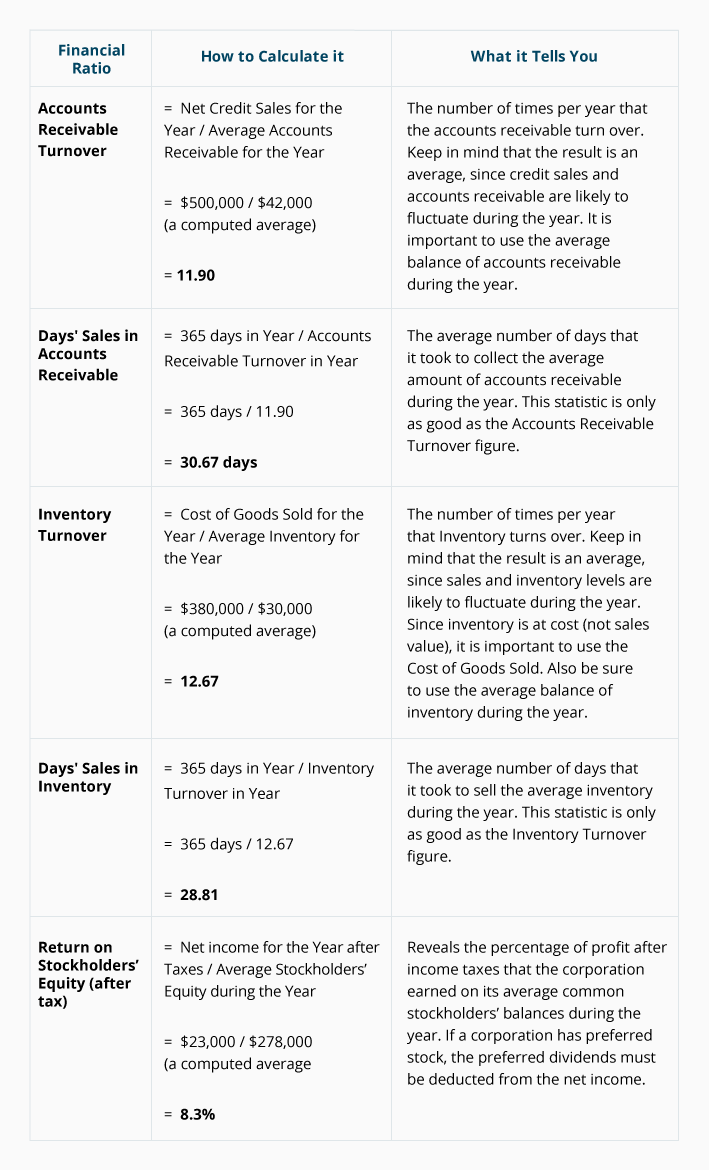### Calculating a Ratio Using Amounts from the Statement of Cash Flows

Use the following statement of cash flows (SCF) for the related ratio calculation appearing after the SCF: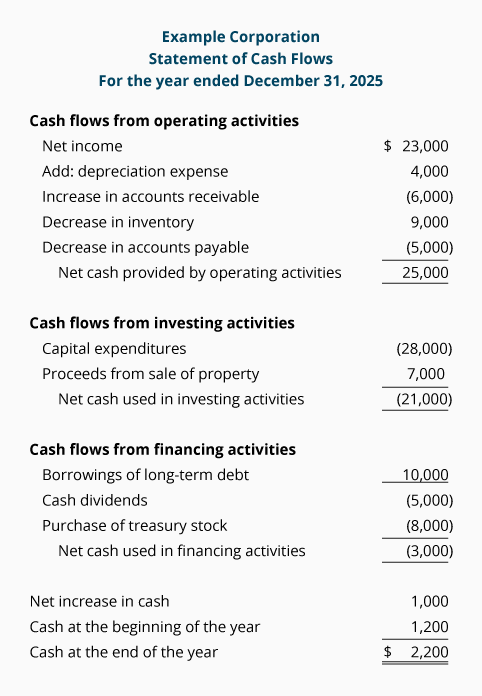Calculation of Free Cash Flow
Using Example Corporation's statement of cash flows (above), the amount of the corporation's free cash flow for the year 2022 was ________________.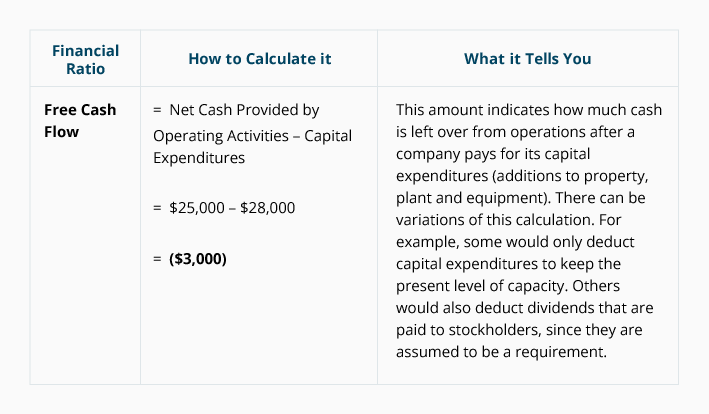#### Take Our Practice Quiz

We recommend that you now take our free Practice Quiz for this topic so that you can...

• See what you know
• See what you don't know